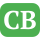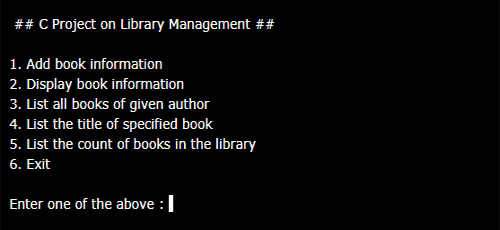CppBuzz.com

# C Project on Library Management

C project on library management with options to Add books, List Books, List by title, Count books etc.. Program uses structure to store books records.

## Source Code

```/*
Program: Library Management
Author: CppBuzz
Date: 17th Nov 2017
*/

#include<stdio.h>
#include<conio.h>
#include<stdlib.h>
#include<string.h>

struct library
{
char bk_name;
char author;
int pages;
float price;
};

int main()
{
struct library l;
char ar_nm,bk_nm;
int i,j, keepcount;
i=j=keepcount = 0;

while(j!=6)
{
printf("\n\n1. Add book information\n2. Display book information\n");
printf("3. List all books of given author\n");
printf("4. List the title of specified book\n");
printf("5. List the count of books in the library\n");
printf("6. Exit");

printf ("\n\nEnter one of the above : ");
scanf("%d",&j);

switch (j)
{
case 1:

printf ("Enter book name = ");
scanf ("%s",l[i].bk_name);

printf ("Enter author name = ");
scanf ("%s",l[i].author);

printf ("Enter pages = ");
scanf ("%d",&l[i].pages);

printf ("Enter price = ");
scanf ("%f",&l[i].price);
keepcount++;

break;
case 2:
printf("you have entered the following information\n");
for(i=0; i<keepcount; i++)
{
printf ("book name = %s",l[i].bk_name);

printf ("\t author name = %s",l[i].author);

printf ("\t  pages = %d",l[i].pages);

printf ("\t  price = %f",l[i].price);
}
break;

case 3:
printf ("Enter author name : ");
scanf ("%s",ar_nm);
for (i=0; i<keepcount; i++)
{
if (strcmp(ar_nm, l[i].author) == 0)
printf ("%s %s %d %f",l[i].bk_name,l[i].author,l[i].pages,l[i].price);
}
break;

case 4:
printf ("Enter book name : ");
scanf ("%s",bk_nm);
for (i=0; i<keepcount; i++)
{
if (strcmp(bk_nm, l[i].bk_name) == 0)
printf ("%s \t %s \t %d \t %f",l[i].bk_name,l[i].author,l[i].pages,l[i].price);
}
break;

case 5:
printf("\n No of books in library : %d", keepcount);
break;
case 6:
exit (0);

}
}
return 0;

}
```

## Output of Project